# Balbharati solutions for Economics 12th Standard HSC Maharashtra State Board chapter 3 - Elasticity of Demand [Latest edition]

#### Chapters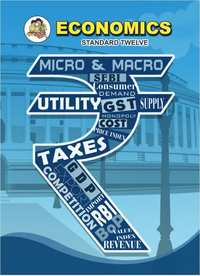## Chapter 3: Elasticity of Demand

Exercise
Exercise [Pages 34 - 36]

### Balbharati solutions for Economics 12th Standard HSC Maharashtra State Board Chapter 3 Elasticity of Demand Exercise [Pages 34 - 36]

Exercise | Q 1. (1) | Page 34

Complete the following statement:

Price elasticity of demand on a linear demand curve at the X-axis is ________.

• zero

• one

• infinity

• less than one

Exercise | Q 1. (2) | Page 34

Complete the following statement:

Price elasticity of demand on a linear demand curve at the Y-axis is equal to ________.

• zero

• one

• infinity

• greater than one

Exercise | Q 1. (3) | Page 34

Complete the following statement:

Demand curve is parallel to X-axis, in the case of ______.

• perfectly elastic demand

• perfectly inelastic demand

• relatively elastic demand

• relatively inelastic demand

Exercise | Q 1. (4) | Page 34

Complete the following statement:

When the percentage change in quantity demanded is more than the percentage change in price, the demand curve is ______.

• flatter

• steeper

• rectangular

• horizontal

Exercise | Q 1. (5) | Page 35

Ed = 0 in case of ______.

• luxuries

• normal goods

• necessities

• comforts

Exercise | Q 2. (1) | Page 35

Give economic term:

Degree of responsiveness of quantity demanded to change in income only.

Exercise | Q 2. (2) | Page 35

Give economic term:

Degree of responsiveness of a change in quantity demanded of one commodity due to change in the price of another commodity.

Exercise | Q 2. (3) | Page 35

Give economic term:

Degree of responsiveness of a change of quantity demanded of a good to a change in its price.

Exercise | Q 2. (4) | Page 35

Give economic term:

Elasticity resulting from infinite change in quantity demanded.

Exercise | Q 2. (5) | Page 35

Give economic term:

Elasticity resulting from a proportionate change in quantity demanded due to a proportionate change in price.

Exercise | Q 3. (1) | Page 35

Complete the correlation:

Perfectly elastic demand : Ed = ∞ :: _______ : Ed = 0

Exercise | Q 3. (2) | Page 35

Complete the correlation:

Rectangular hyperbola : ______ : Steeper demand curve : Relatively inelastic demand.

Exercise | Q 3. (3) | Page 35

Complete the correlation:

Straight-line demand curve : Linear demand curve :: _______ : non linear demand curve.

Exercise | Q 3. (4) | Page 35

Complete the correlation:

Pen and ink : ______ :: Tea and Coffee : Substitutes.

Exercise | Q 3. (5) | Page 35

Complete the correlation:

Ratio method : Ed = (%triangle"Q")/(%triangle"P") :: _______ : Ed = "Lower segment"/"Upper segment"

Exercise | Q 4. (1) | Page 35

Assertion and Reasoning type of question:

Assertion (A): Elasticity of demand explains that one variable is influenced by another variable.

Reasoning (R): The concept of elasticity of demand indicates the effect of price and changes in other factors on demand.

• (A) is true, but (R) is false

• (A) is false, but (R) is true

• Both (A) and (R) are true and (R) is the correct explanation of (A)

• Both (A) and (R) are true and (R) is not the correct explanation of (A)

Exercise | Q 4. (2) | Page 35

Assertion and Reasoning type of question:

Assertion (A): A change in quantity demanded of one commodity due to a change in the price of other commodity is cross elasticity

Reasoning (R): Changes in consumer income leads to a change in the quantity demanded.

• (A) is true, but (R) is false

• (A) is false, but (R) is true

• Both (A) and (R) are true and (R) is the correct explanation of (A)

• Both (A) and (R) are true and (R) is not the correct explanation of (A)

Exercise | Q 4. (3) | Page 35

Assertion and Reasoning type of question:

Assertion (A): Degree of price elasticity is less than one in case of relatively inelastic demand.

Reasoning (R): Change in demand is less than the change in price.

• (A) is true, but (R) is false

• (A) is false, but (R) is true

• Both (A) and (R) are true and (R) is the correct explanation of (A)

• Both (A) and (R) are true and (R) is not the correct explanation of (A)

Exercise | Q 5. (1) | Page 35

Distinguish between Relatively elastic demand and relatively inelastic demand.

Exercise | Q 5. (2) | Page 35

Distinguish between Perfectly elastic demand and perfectly inelastic demand.

Exercise | Q 6. (1) | Page 35

What is ‘elasticity of demand’? Explain the factors determining elasticity of demand.

Exercise | Q 6. (2) | Page 35

Explain the total outlay method of measuring elasticity of demand?

Exercise | Q 6. (3) | Page 35

Explain the importance of elasticity of demand.

Exercise | Q 7. (1)(a) | Page 36

Identify and define the degrees of elasticity of demand from the following demand curve.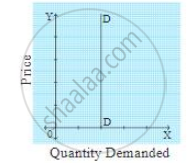Exercise | Q 7. (1)(b) | Page 36

Identify and define the degrees of elasticity of demand from the following demand curve.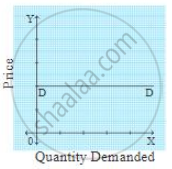Exercise | Q 7. (1)(c) | Page 36

Identify and define the degrees of elasticity of demand from the following demand curve.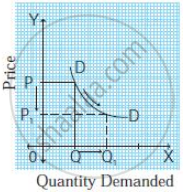Exercise | Q 7. (1)(d) | Page 36

Identify and define the degrees of elasticity of demand from the following demand curve.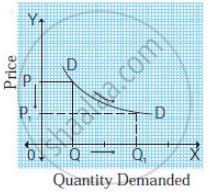Exercise | Q 7. (2)(1) | Page 36

In the following diagram, AE is the linear demand curve of a commodity. On the basis of the given diagram state whether the following statement is True or False. Give a reason for your answer.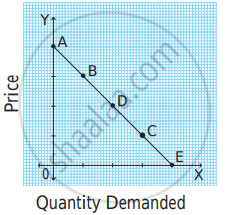Demand at point ‘C’ is relatively elastic demand.

• True

• False

Exercise | Q 7. (2)(2) | Page 36

In the following diagram, AE is the linear demand curve of a commodity. On the basis of the given diagram state whether the following statement is True or False. Give a reason for your answer.Demand at point ‘B’ is unitary elastic demand.

• True

• False

Exercise | Q 7. (2)(3) | Page 36

In the following diagram, AE is the linear demand curve of a commodity. On the basis of the given diagram state whether the following statement is True or False. Give a reason for your answer.Demand at point ‘D’ is perfectly inelastic demand.

• True

• False

Exercise | Q 7. (2)(4) | Page 36

In the following diagram, AE is the linear demand curve of a commodity. On the basis of the given diagram state whether the following statement is True or False. Give a reason for your answer.Demand at point ‘A’ is perfectly elastic demand.

• True

• False

## Chapter 3: Elasticity of Demand

Exercise## Balbharati solutions for Economics 12th Standard HSC Maharashtra State Board chapter 3 - Elasticity of Demand

Balbharati solutions for Economics 12th Standard HSC Maharashtra State Board chapter 3 (Elasticity of Demand) include all questions with solution and detail explanation. This will clear students doubts about any question and improve application skills while preparing for board exams. The detailed, step-by-step solutions will help you understand the concepts better and clear your confusions, if any. Shaalaa.com has the Maharashtra State Board Economics 12th Standard HSC Maharashtra State Board solutions in a manner that help students grasp basic concepts better and faster.

Further, we at Shaalaa.com provide such solutions so that students can prepare for written exams. Balbharati textbook solutions can be a core help for self-study and acts as a perfect self-help guidance for students.

Concepts covered in Economics 12th Standard HSC Maharashtra State Board chapter 3 Elasticity of Demand are Elasticity of Demand, Types of Elasticity of Demand, Types of Price Elasticity of Demand, Methods of Measuring Price Elasticity of Demand, Factors Influencing the Elasticity of Demand, Importance of Elasticity of Demand, Importance of Price Elasticity of Demand.

Using Balbharati 12th Board Exam solutions Elasticity of Demand exercise by students are an easy way to prepare for the exams, as they involve solutions arranged chapter-wise also page wise. The questions involved in Balbharati Solutions are important questions that can be asked in the final exam. Maximum students of Maharashtra State Board 12th Board Exam prefer Balbharati Textbook Solutions to score more in exam.

Get the free view of chapter 3 Elasticity of Demand 12th Board Exam extra questions for Economics 12th Standard HSC Maharashtra State Board and can use Shaalaa.com to keep it handy for your exam preparation